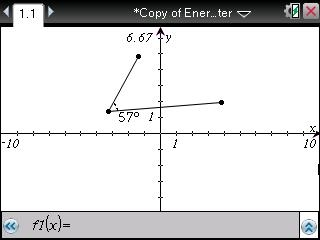# Knowledge Base

## Solution 31891: Calculating Measurements for Length, Area, Slope, and Angles Using the TI-Nspire™ Family Handhelds.

### How do I calculate measurements for length, area, slope, and angles using the TI-Nspire family handhelds?

The TI-Nspire family handhelds can calculate the measurements for length, area, slope, and angles of figures. To access the measurements options you will first need to insert a graphs application. Please follow the steps below:

• Press [home].
• Select 8: Geometry.
• Select 3: Measurement.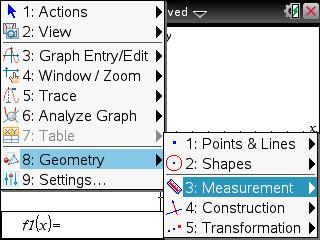Length

Length is used to calculate the length of line segments or the sides of shapes. To find the length option, please follow the steps below:

• Select 8: Geometry.
• Select 3: Measurement .
• Select 1: Length.

To find the length, move the cursor and press [enter] to select the line that you want to find the length of. You can then move the cursor to the desired area and press [enter] again to set the length value.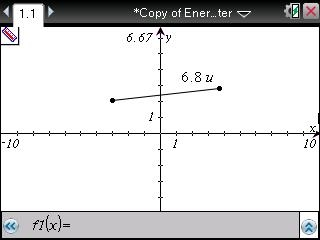Area

Area is used to find the area within shapes, such as squares and circles.

• Select 8: Geometry.
• Select 3: Measurement.
• Select 2: Area.

To find the area, move the cursor and press [enter] tp select the shape that you want to find the area of. You can then move the cursor to the desired area and press [enter] again to set the area value.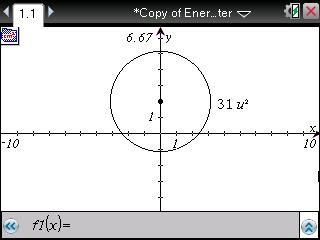Slope

Slope is used to find the slope of a line. It cannot be used to find the slope of curved lines, such as parabolas.

• Select 8: Geometry.
• Select 3: Measurement.
• Select 3: Slope.

To find the slope, move the cursor and press [enter] to select the line that you want to find the slope of. You can then move the cursor to the desired area and press [enter] again to set the slope value.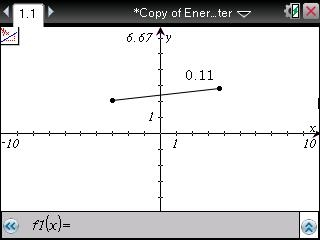Angle

Angle is used to find the angle between two lines. You can either choose from existing lines on the graph or create new ones. It can display the answer in radians or degrees, depending on your mode setting.